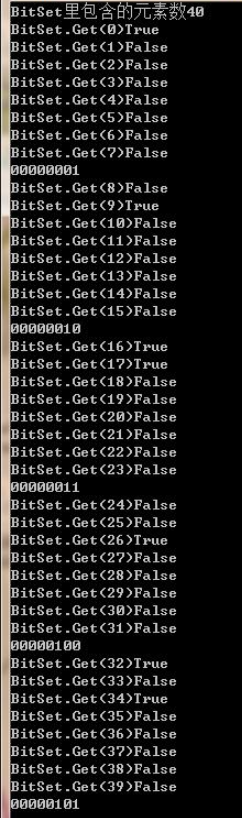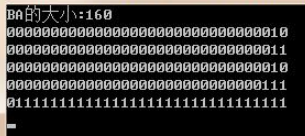﻿

## 一、BitConverter 将预定义的基础类型与字节数据进行互转（Unicode）

### 1、将值类型转成字节数组(Unicode)：BitConverter.GetBytes()

byte[] data = BitConverter.GetBytes('哈');
Console.Write(data);//200,84 （两个字节）
Console.Write(BitConverter.GetBytes('1'));//49,0 （两个字节）
Console.Write(BitConverter.GetBytes(258));//2,1,0,0  (整数，32位，四个字节)
Console.Write(BitConverter.GetBytes(1.2));//8个元素 （八个字节）
Console.Write(BitConverter.GetBytes(true));//1 （一个字节）


### 2、将字节数组转成值类型：BitConverter.ToXXX()

char str = BitConverter.ToChar(new byte[] { 200, 84 }, 0);
Console.Write(str);//哈
short sho = BitConverter.ToInt16(new byte[] { 69, 0 }, 0);
Console.Write(sho.ToString());//69

### 3、将字节数组中每个元素值转成十六进制表示：BitConverter.ToString（byte[]）

string strHex = BitConverter.ToString(new byte[] { 09, 45, 65, 0, 0 });
Console.Write(strHex);//09-2D-41-00-00
string strHex = BitConverter.ToString(new byte[] { 69, 0, 0, 0, 0 });
Console.Write(strHex);//45-00-00-00-00

### 4、举例说明：

 整数 对应的十六进制数 (Convert.ToString(**, 16) ) 转成字节数组byte[] BitConverter.GetBytes(**) 字节数据每个字节对应的十六进制表示 BitConverter.ToString(**) 1 1：0x01 (两位两位地对齐) [1,0,0,0] 01- 00- 00 -00 65540 10004：0x1 00 04 (两位两位地对齐) [4,0,1,0] 04- 00- 01 -00 999999999 3B9AC9FF：0x3B9AC9FF (两位两位地对齐) [255,201,154,59] FF -C9- 9A -3B

## 二、字符与ASCII码转化：

### 1、字符转成ASCII码：

Console.Write(Convert.ToByte('a'));//97
Console.Write((int)'a');//97，强制转换
Console.Write(Convert.ToByte('1'));//49
Console.Write((int)'1');//49

### 2、ASCII码转成字符：

Convert.ToChar(97)=>’d’;  (char)97=’a’ --强制转换：

## 三、字符串与十六进制表示的字节数组之间的转换

void Main()
{
Console.WriteLine(StrToHex("哈啊a的1我z!"));
Console.WriteLine(HexToStr("B9 FE B0 A1 61 B5 C4 31 CE D2 7A 21"));
}

//字符串转换成十六进制字符串
public string StrToHex(string mStr)
{
return BitConverter.ToString(ASCIIEncoding.Default.GetBytes(mStr)).Replace("-", " ");
}
// 十六进制字符串转换成字符串
public string HexToStr(string mHex)
{
mHex = mHex.Replace(" ", "");
if (mHex.Length <= 0) return "";
byte[] vBytes = new byte[mHex.Length / 2];
for (int i = 0; i < mHex.Length; i += 2)
if (!byte.TryParse(mHex.Substring(i, 2), NumberStyles.HexNumber, null, out vBytes[i / 2]))
vBytes[i / 2] = 0;
return ASCIIEncoding.Default.GetString(vBytes);
}

## 四、BitArray类

BitArray类用来处理位集合。位集合是可以用来有效表示Boolean值的集合。注意：表示True/False的集合，不是0、1集合。

0 0 0 0 0 0 0 1

True False False False False False False False

### 属性

• Count 获取 BitArray 中包含的元素个数。
• Item 获取或设置 BitArray 中指定位置的位的值。
• Length 获取或设置 BitArray 中的元素个数。

### 方法

• BitArray And( BitArray value ); 对当前的 BitArray 中的元素和指定的 BitArray 中的相对应的元素执行按位与操作。
• bool Get( int index ); 获取 BitArray 中指定位置的位的值。
• BitArray Not();把当前的 BitArray 中的位值反转，以便设置为 true 的元素变为 false，设置为 false 的元素变为 true。
• BitArray Or( BitArray value ); 对当前的 BitArray 中的元素和指定的 BitArray 中的相对应的元素执行按位或操作。
• void Set( int index, bool value ); 把 BitArray 中指定位置的位设置为指定的值。
• void SetAll( bool value ); 把 BitArray 中的所有位设置为指定的值。
• BitArray Xor( BitArray value ); 对当前的 BitArray 中的元素和指定的 BitArray 中的相对应的元素执行按位异或操作。
• CopyTo(Array, Int32) 从目标数组的指定索引处开始将整个 BitArray 复制到兼容的一维 Array。

### 举例：

int bits;
string[] binNumber = new string;//
int binary;
byte[] ByteSet = new byte[] { 1, 2, 3, 4, 5 };//定义一个字节型数组，存5个字节型数值：1,2,3,4,5,6
BitArray BitSet = new BitArray(ByteSet);//这里一定要注意，这里的每一个字节型数值是以位的形式、每一位用Boolean值、逆序存储在BitArray（1字节对应8位）
bits = 0;//bits是计数器，到8了就又归为初始值0；为的是方便知道操作完了一个字节型数值（1字节正好占8位）
binary = 7;//binary是二进制数组下标索引；初始值为7，这是因为BitArray里存储的数据和常规二进制数据的顺序是相反的
Console.WriteLine("BitSet里包含的元素数" + BitSet.Count);//40，因为数据在BitArray里是以位的形式存储的，5个字节型数值，一个字节占8位，所以共占40位！！！不是5！！！
for (int i = 0; i <= BitSet.Count - 1; i++)//遍历40个位，每个位存boolean值True/False
{
Console.WriteLine("BitSet.Get(" + i + ")" + BitSet.Get(i));
if (BitSet.Get(i) == true)
binNumber[binary] = "1";//如果该位存储的true值，则转成1；
else
binNumber[binary] = "0";//如果该位存储的false值，则转成0；
bits++;      //计数+1
binary--;    //eg.第8位（下标7）存好，则将下标向左移1位存下一轮将位布尔值转成的二进制0/1

if ((bits % 8) == 0)//当计数器为8时，表示一个字节型的数值已经表示完全了
{
binary = 7;//将存储字节型数值的二进制形式的String型数组的下标归初始
bits = 0;
for (int j = 0; j <= 7; j++)
Console.Write(binNumber[j]);
Console.WriteLine();
}
}int bits = 0;
int index = 31;
string[] s = new string;
int[] a = new int[] { 2, 3, 2, 7, 2147483647 };
BitArray BA = new BitArray(a);

Console.WriteLine("BA的大小:" + BA.Count);  //5*4*8=160

for (int i = 0; i < BA.Count; i++)
{
Boolean b = BA.Get(i);
if (b == true)
s[index] = "1";
else
s[index] = "0";
bits++;
index--;
if (bits % 32 == 0) //每隔一个数字（4*8）换行
{
bits = 0;
index = 31;
for (int j = 0; j <= 31; j++)
Console.Write(s[j]);
Console.WriteLine();
}
}posted on 2018-08-28 10:48  springsnow  阅读(654)  评论(0编辑  收藏